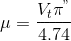# Galactic Rotation and Gas Clouds

• JazzCarrot

## Homework Statement

Two questions here, but it's mainly terminology I don't understand;

1(a). Calculate the proper motion of the Galactic Centre (GC) with respect
to the sun (and measured relative to the ‘stationary’ distant galaxies
and quasars) in units of arcsec/yr, assuming that the Galaxy rotation
speed at the solar circle is Vo = 220 km/s and the distance to the GC
is Ro = 8.5 kpc.

2. A spherical HI cloud has a density of 106 atoms m-3 and a gas mass of
50 M⊙
(a). What is the cloud radius in pc?
(b). Show that the luminosity of the cloud in its 1.42 GHz (21 cm) emission
line is 1.6×1020 W.
(c). Assume the cloud lies at a distance of 4 kpc in a direction l = 0o and is
observed with a radio telescope of field of view (beam-size) 20 arcmin.
What flux would you measure from the cloud in its 1.42 GHz (21 cm)
emission line?
(d). Random bulk motions in the cloud of ±10 km/s along the line of
sight Doppler-broaden the observed line. What is the full width in
(c) write down the average flux per unit frequency, and express your
answer in units of Janskys (Jy), where 1 Jy = 10−26W/m2/Hz.

2. Homework Equations

Question 1 -I'm not sure how to get Vt

3. The Attempt at a Solution

1. Galaxy rotation speed at the solar circle is Vo = 220 km/s

I'm confused at what this speed actually represents. For the equation of proper motion, I need the transverse velocity, Vt. Which means I might have to decompose Vo into components Vt and Vr using an angle... which I don't know2. c) and is observed with a radio telescope of field of view (beam-size) 20 arcmin.

I'm not sure how to relate the beam-size into measuring the flux, other than I assume it will be related to the proportion of the flux it can measure compared to amount received at Earth (Which I've found to be 8.3x10-22W/m2).

Any help is greatly appreciated.

1. Galaxy rotation speed at the solar circle is Vo = 220 km/s

I'm confused at what this speed actually represents. For the equation of proper motion, I need the transverse velocity, Vt. Which means I might have to decompose Vo into components Vt and Vr using an angle... which I don't knowIt's a circle. Isn't the velocity always tangential at any instant? I mean, assuming that we are actually orbiting in a perfect circle of radius 8.5 kpc around the centre of the galaxy, what that means is that the centre of the galaxy's distance to us is never changing. It is never getting closer to or farther away from us. So how could its velocity possibly have a radial component?

2. c) and is observed with a radio telescope of field of view (beam-size) 20 arcmin.

I'm not sure how to relate the beam-size into measuring the flux, other than I assume it will be related to the proportion of the flux it can measure compared to amount received at Earth (Which I've found to be 8.3x10-22W/m2).

Although, to be honest, I don't even know how to solve part (b), assuming that you can, then I guess my starting point for part (c) would be to ask, what angle does the HI region subtend at that distance, and how does this compare to the field of view? Then, at least you know how much of the cloud your telescope is seeing.